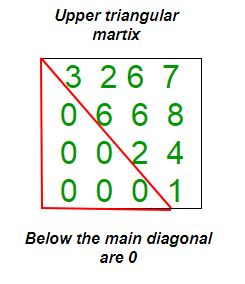GeeksforGeeks App
Open AppBrowser
Continue

# Java Program to check if matrix is upper triangular

Given a square matrix and the task is to check the matrix is in upper triangular form or not. A square matrix is called upper triangular if all the entries below the main diagonal are zero.Examples:

```Input : mat = {{1, 3, 5, 3},
{0, 4, 6, 2},
{0, 0, 2, 5},
{0, 0, 0, 6}};
Output : Matrix is in Upper Triangular form.

Input : mat = {{5, 6, 3, 6},
{0, 4, 6, 6},
{1, 0, 8, 5},
{0, 1, 0, 6}};
Output : Matrix is not in Upper Triangular form.```

## Java

 `// Java Program to check upper``// triangular matrix.``import` `java.util.*;``import` `java.lang.*;` `public` `class` `GfG``{``    ``private` `static` `final` `int` `N = ``4``;` `    ``// Function to check matrix is in``    ``// upper triangular form or not.``    ``public` `static` `Boolean isUpperTriangularMatrix(``int` `mat[][])``    ``{``        ``for` `(``int` `i = ``1``; i < N ; i++)``            ``for` `(``int` `j = ``0``; j < i; j++)``                ``if` `(mat[i][j] != ``0``)``                    ``return` `false``;``        ``return` `true``;``    ``}``    ` `    ``// driver function``    ``public` `static` `void` `main(String argc[]){``        ``int``[][] mat= { { ``1``, ``3``, ``5``, ``3` `},``                       ``{ ``0``, ``4``, ``6``, ``2` `},``                       ``{ ``0``, ``0``, ``2``, ``5` `},``                       ``{ ``0``, ``0``, ``0``, ``6` `} };``                    ` `        ``if` `(isUpperTriangularMatrix(mat))``            ``System.out.println(``"Yes"``);``        ``else``            ``System.out.println(``"No"``);``    ``}``}` `/* This code is contributed by Sagar Shukla */`

Output:

`Yes`

Time Complexity: O(n2), where n represents the number of rows and columns of the matrix.
Auxiliary Space: O(1), no extra space is required, so it is a constant.

Please refer complete article on Program to check if matrix is upper triangular for more details!

My Personal Notes arrow_drop_up